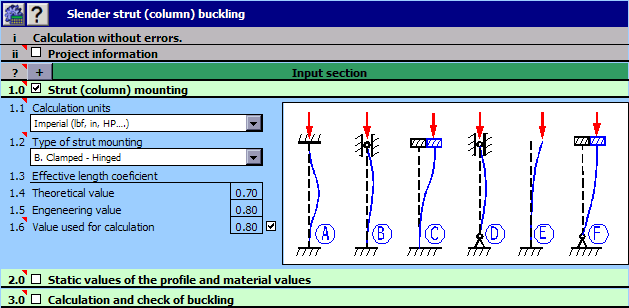MITCalc - Buckling Calculation Description

Calculation of the optimum cross-section of slender struts strained for buckling and calculation of the appropriate
strength check according to Johnson, Tetmajer, Euler and Secant method. Simple definition of the strut type - 40
types of predefined cross sections.Used standards: DIN 1025, 1026, 1028, 1029, 1024, AISC W, S, C, L, LU

Category: Education And Reference > Math

Size: 1525KB

OS: Win 95/98/Me/NT/2000/XPTalking Math Tutor

This is the perfect Program for students to practice their Math skills. The program Talks and Works with the student one on one.

Percent

Calculate the percentage of any number out of any number. Add percentage to an amount or calculate a discount percentage. Free math homework help from basic math to algebra, geometry and beyond. Students, teachers, parents, and everyone can find solutions

Visual Data

Creating graphs of regular data and irregular data. Creating vertex, grid and surface model, color map, contour plot, vector plot, 4D scatter, 4D slice and 4D vector.